Do you like the article?
Share it with others -
Use new possibilities of MetaTrader 5

# Creating Custom Criteria of Optimization of Expert Advisors

7 September 2011, 12:23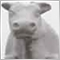12
17 817

### Introduction

The MetaTrader 5 Client Terminal offers a wide range of opportunities for optimization of Expert Advisor parameters. In addition to the optimization criteria included in the strategy tester, developers are given the opportunity of creating their own criteria. This leads to an almost limitless number of possibilities of testing and optimizing of Expert Advisors. The article describes practical ways of creating such criteria - both complex and simple ones.

### 1. The Review of Features of the Strategy Tester

This subject has been discussed many times, so I'm only going to make a list of articles with their short descriptions. I recommend you to get acquainted with the following materials before reading this article.

And of course, at first you need to get acquainted with the documentation provided with the client terminal.

### 2. The Optimization Criteria Embedded in the Strategy Tester

If you look in the documentation, you'll find the following description: Optimization criterion is a certain factor, whose value defines the quality of a tested set of parameters. The higher the value of the optimization criterion is, the better the testing result with the given set of parameters is considered to be.

Here we should make an important note: an optimization criterion can be used only in the genetic algorithm mode of optimization. It is clear, that when going over all possible combination of parameter values, there cannot be any factor of choosing optimal parameters of an Expert Advisor. The other side is we can save the results of testing and then process them to find an optimal combination of parameters.

As is written in the documentation, the strategy tester includes the following optimization criteria to be used with the genetic algorithm:

• Balance max - the highest value of the balance;
• Balance + max Profit Factor - the highest value of the product of balance and profit factor;
• Balance + max Expected Payoff - the value of the product of balance and the expected payoff;
• Balance + min Drawdown - in this case, the balance value and the drawdown level are taken into account: (100% - Drawdown)*Balance;
• Balance + max Recovery Factor - the product of the balance and the recovery factor;
• Balance + max Sharpe Ratio - the value of the product of balance and the Sharpe ratio;
• Custom max - custom criterion of optimization. The optimization criterion here is the value of the OnTester() function in the Expert Advisor. This parameter allows using any custom value for the optimization of the Expert Advisor.

An optimization criteria can be selected on the Settings tab of the strategy tester as is shown in the fig. 1: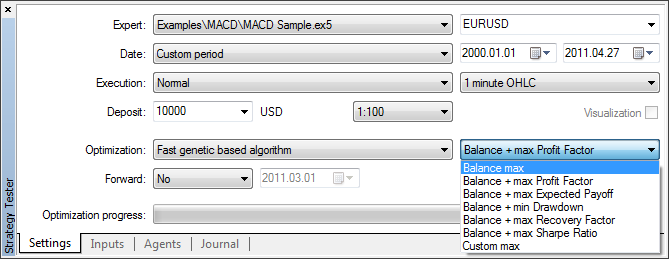Fig. 1. Choosing optimization criterion for Expert Advisor

The Custom max criterion, which is last in the list , is the most interesting for us, and its usage is the subject of this article.

### 3. Creation of Custom Optimization Criteria

The first thing that should be done is giving a user the possibility of free combination of parameters (not limited to the ones shown in the fig. 1, but custom), that are calculated by the strategy tester after each run of an Expert Advisor.

For example, the following variant is interesting: Balance max + min Drawdown + Trades Number - the more trades there are, the more reliable is the result. Or the following one - Balance max + min Drawdown + max Profit Factor. Of course, there are a lot of other interesting combinations that are not included in the strategy tester settings.

Let's call such combinations of criteria as simple criteria of optimization.

But those criteria are not enough to make a reliable estimation of a trade system. If we look from the trading concept point of view - making a profit at minimal risk- we can assume the following criterion: we may optimize parameters to get a smoothest curve of balance with minimal deviation of results of separate trades from the straight line.

Let's call this criterion as criterion of optimization by balance curve.

The next criterion of optimization we're going to use is the coefficient of safety of a trade system. This coefficient is described in the "Be In-Phase" article. It characterizes the correspondence of a trade system to the market; that is what we need to find out during optimization of parameters. Let's call it as criterion of optimization by the coefficient of safety of a trade system (CSTS).

In addition, let's make it possible to combine the described criteria freely.

### 4. The OnTester() Function

Before writing the code parts, let's take a look at organization of usage of custom criteria of EA optimization in the strategy tester.

The predefined function OnTester() is intended for creation of custom criteria of optimization. It is automatically called at the end of each pass of testing of an Expert Advisor within a specified time range. This function is called right before the call of the OnDeinit() function.

Once again, pay attention that to use the OnTester() function, you should enable the Fast genetic base algorithm mode of optimization as is shown in the fig.1.

This function has the double format of returned value, which is used for optimization in the strategy tester.

Take a look at the documentation once again:

In the genetic optimization descending sorting is applied to results within one generation. I.e. from the point of view of the optimization criterion, the best results are those with largest values. In such a sorting, the worst values are positioned at the end and further thrown off and do not participate in the forming of the next generation.

Thus, when creating a custom optimization criterion, we need to get an integral value that will be used for estimation of trading of the Expert Advisor. The greater the value is, the better is trading of the Expert Advisor.

### 5. Writing Experimental Expert Advisor

Now it's time to make an Expert Advisor that we're going to optimize in the strategy tester. In this case, the main requirements for it are being simple and fast not to spare a lot of time for the routine procedure of optimization. Also, it is desirable if the Expert Advisor is not very unprofitable.

Let's take the Expert Advisor described in the "Several Ways of Finding a Trend in MQL5" article as the experimental one and improve it. Notably, the EA based on the "fan" of three moving averages. The improvement consists in getting rid of using of the indicator to increase the speed of operation and moving the calculation part of the code inside the EA itself. This allows increasing the speed of testing significantly (almost three times at a two-year range).

The part of setting input parameters is simple:

```input double Lots = 0.1;
input int  MA1Period = 200; // period of the greatest moving average
input int  MA2Period = 50;  // period of the medium moving average
input int  MA3Period = 21;  // period of the smallest moving average```

The periods of the moving averages are what we are going to optimize.

The structure and operation of the Expert Advisor are described in details in the article mentioned above, so let's skip it here. The main innovation is the handler of the event of completion of another test pass - the OnTester() function. Currently, it is empty and returns the control.

```//---------------------------------------------------------------------
//  The handler of the event of completion of another test pass:
//---------------------------------------------------------------------
double OnTester()
{
return(0.0);
}```

The file of the EA - FanExpert.mq5 is attached to this article. We can make sure that is identical to the FanTrendExpert.mq5 EA from the performed deals point of view. The check of existence and direction of a signal is performed at opening of a new bar on a chart.

To get the result of testing calculated at the end of each pass, the TesterStatistics() is used; it returns the requested statistical value calculated as a result of testing. It can be called only from the OnTester() and OnDeinit() function, otherwise the result is undefined.

Now, let's add a custom optimization criterion. Suppose that we need to find optimal results on the basis of a maximal value of recovery factor - max Recovery Factor. To do it, we need to know the values of the max. drawdown of balance in money and the gross profit at the end of testing. The recovery facto is calculated as division of the profit on the maximal drawdown.

It is done just as an example, since the recovery factor is already included is in the list of calculated statistical results of testing.

To do it, add the following simple code to the OnTester() function:

```//---------------------------------------------------------------------
//  The handler of the event of completion of another test pass:
//---------------------------------------------------------------------
double OnTester()
{
double  profit = TesterStatistics(STAT_PROFIT);
double  max_dd = TesterStatistics(STAT_BALANCE_DD);
double  rec_factor = profit/max_dd;

return(rec_factor);
}```

The check for zero divide is excluded from the code to make it easier. Since the maximal drawdown can be equal to zero, this check must be done in a real Expert Advisor.

Now, let's create the criterion mentioned above: Balance max + min Drawdown + Trades Number - Balance + Minimal Drawdown + Number of Trades.

To do it, change the OnTester() function in the following way:

```double OnTester()
{
double  param = 0.0;

//  Balance max + min Drawdown + Trades Number:
double  balance = TesterStatistics(STAT_PROFIT);
double  min_dd = TesterStatistics(STAT_BALANCE_DD);
if(min_dd > 0.0)
{
min_dd = 1.0 / min_dd;
}
param = balance * min_dd * trades_number;

return(param);
}```

Here we take a value that is opposite to the drawdown, because the smaller the drawdown is, the better is the situation, supposing that other conditions are equal. Run the optimization of the FanExpert EA with the created optimization criterion by the MA1Period parameter using the 2009.06.01 - 2011.06.03 range and the Н1 timeframe. Set the range of values of the moving average from 100 to 2000.

At the end of optimization you'll get the following table of values sorted by the best parameters: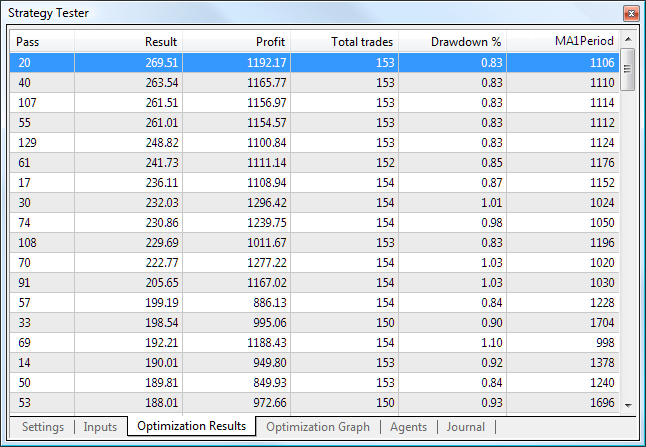Fig. 2. The best results of optimization by the Balance max + min Drawdown + Trades Number criterion

The best parameters are listed here (by the Result column).

Now, let's take a look at the worst parameters: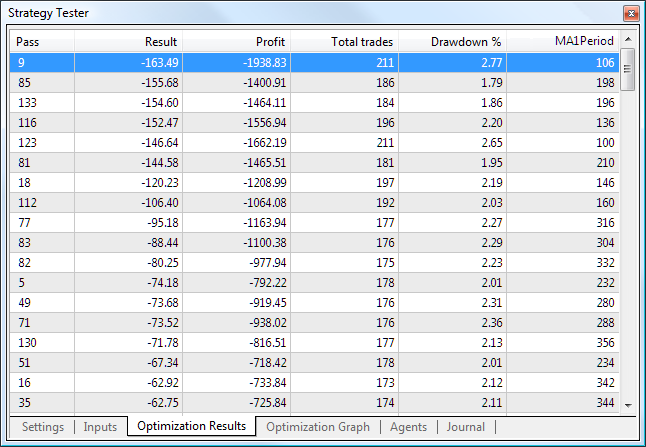Fig. 3. The worst parameters of optimization by the Balance max + min Drawdown + Trades Number criterion

Comparing two tables, you can see that the drawdown and the profit are considered along with the number of trades, i.e. our optimization criterion is working. In addition, we can see the optimization graph (linear):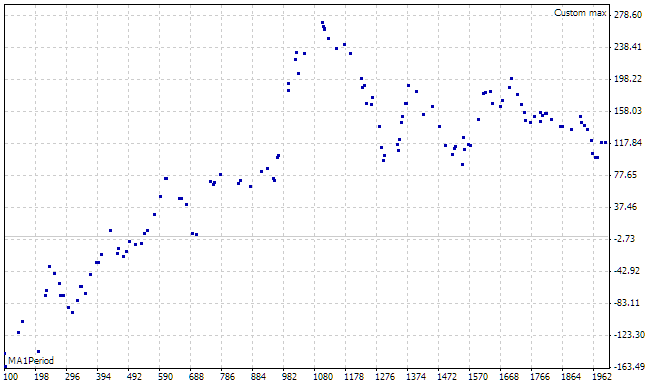Fig. 4. The graph of optimization by the Balance max + min Drawdown + Trades Number criterion

The horizontal axis displays the optimized parameter, and the vertical one displays the optimization criterion. We can see the clear maximum of the set criterion; it is located within the 980 to 1200 range of periods.

You should understand and remember that it is the genetic optimization of parameters, not the full search. That's why the tables shown in the fig. 2 and fig. 3 contain the most "viable" parameters that have passed the natural selection in several generations. Probably, some successful variants have been discarded.

The balance/equity curve for the 1106 period looks as following: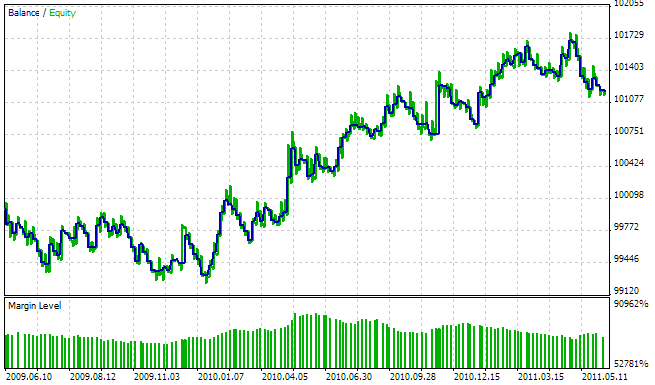Fig. 5. The balance/equity curve for the MA1Period = 1106 period

### 6. Creation of Classes of Custom Optimization Criteria

So, we've learned how to create and used simple optimization criteria. Now, let's make a class to simplify their usage in Expert Advisors. One of the main requirements for such class is the speed of operation in addition to the convenience of use. Calculations of optimization criteria must be performed quickly, otherwise you'll wait long for the results.

MetaTrader 5 allows using the technology of cloud calculation for the optimization. This is a huge breakthrough, since the processing of a great number of parameters requires gigantic calculation power. Thus, for developing our class we're going to use the most simple and fast solutions, even though they're not so elegant from the programming point of view.

For the development, we're going to use the standard classes of organization of data that are delivered together with the client terminal.

First of all, let's classify the types of calculated statistical results of testing:

• Floating or integer type with the direct proportionality between the values of testing result and optimization criterion.

In other words, the greater is the value of the result of testing, the better and greater is the value of the optimization criterion. A striking example of such result of testing is the Gross profit at the end of testing STAT_PROFIT. The value has the floating format and can change from negative infinity (actually it is limited by the deposit value) to positive infinity.

Another example of the result of testing of this type is the Number of trades STAT_TRADES. Generally, the greater is the number of trades, the more reliable is the result of optimization. The value has the integer format and can change from zero to positive infinity.

• Floating or integer type with the inverse proportionality between the values of testing result and optimization criterion.

In other words, the smaller is the value of the result of testing, the better and greater is the value of the optimization criterion. An example of such result of testing is the Maximum drawdown of balance in money STAT_BALANCE_DD as well as any other drawdown.

To obtain this type of testing result, we're going to take a reverse value for calculation of value of the optimization criterion. Of course, we need to implement the check for zero divide to avoid the corresponding error.

The base class for creation of the custom criteria of optimization TCustomCriterion is very simple. Its purpose is determination of base functionality. It looks as following:

```class TCustomCriterion : public CObject
{
protected:
int     criterion_level;        // type of criterion

public:
int   GetCriterionLevel();
virtual double  GetCriterion();  // get value of the result of optimization
};```

The virtual method TCustomCriterion::GetCriterion should be overridden in inherited classes. This is the main method that returns the value of integral result of testing of an Expert Advisor at the end of each test pass.

The TCustomCriterion::criterion_level class member stores the type of custom criterion inherent in this class instance. It will be used further for differentiation of objects by their types.

Now, we can inherit from it all the classes required for optimization.

The TSimpleCriterion class is intended for creation of "simple" custom criterion that corresponds to a specified statistical result of testing. Its determination looks as following:

```class TSimpleCriterion : public TCustomCriterion
{
protected:
ENUM_STATISTICS  stat_param_type;

public:
ENUM_STATISTICS  GetCriterionType();     // get type of optimized stat. parameter

public:
virtual double   GetCriterion(); // receive optimization result value
TSimpleCriterion(ENUM_STATISTICS _stat); // constructor
};```

Here we use a constructor with parameters; it is implemented as following:

```//---------------------------------------------------------------------
//  Constructor:
//---------------------------------------------------------------------
TSimpleCriterion::TSimpleCriterion(ENUM_STATISTICS _stat)
:
stat_param_type( _stat )
{
criterion_level = 0;
}```

This new feature in the MQL5 language is convenient to use when creating class instances. Also, we've overridden the virtual method TSimpleCriterion::GetCriterion that is used for getting the result of optimization at the end of each test pass. Its implementation is simple:

```//---------------------------------------------------------------------
//  Get the result of optimization:
//---------------------------------------------------------------------
double  TSimpleCriterion::GetCriterion()
{
return(TesterStatistics(stat_param_type));
}```

As you see, it just returns the corresponding statistical result of testing.

The next type of the "simple" custom criterion of optimization is created using the TSimpleDivCriterion class. It is intended for criteria with inverse proportionality between the values of testing result and optimization criterion.

The TSimpleDivCriterion::GetCriterion method looks as following:

```//---------------------------------------------------------------------
//  Get value of the optimization result:
//---------------------------------------------------------------------
double  TSimpleDivCriterion::GetCriterion()
{
double  temp = TesterStatistics(stat_param_type);
if(temp>0.0)
{
return(1.0/temp);
}
return(0.0);
}```

This code doesn't require any additional description.

Two other types of "simple" custom criteria of optimization are created using the TSimpleMinCriterion and TSimpleMaxCriterion classes. They are intended for creation of criteria with limited values of statistical result of testing both from the bottom and the top, respectively.

They can be useful in case you need to discard deliberately wrong values of parameters during optimization. For example, you can limit the minimal number of trades, the maximal drawdown, etc.

The description of the TSimpleMinCriterion class looks as following:

```class TSimpleMinCriterion : public TSimpleCriterion
{
double  min_stat_param;

public:
virtual double  GetCriterion();    // receive optimization result value
TSimpleMinCriterion(ENUM_STATISTICS _stat, double _min);
};```

Here we use the constructor with two parameters. The _min parameter sets the minimum value of a statistical result of testing. If another test pass results in obtaining a values that is less than the specified one, the result is discarded.

The implementation of the TSimpleMinCriterion ::GetCriterion method is following:

```//---------------------------------------------------------------------
//  Get value of the optimization result:
//---------------------------------------------------------------------
double  TSimpleMinCriterion::GetCriterion()
{
double  temp = TesterStatistics(stat_param_type);
if(temp<this.min_stat_param)
{
return(-1.0);
}
return(temp);
}```

The TSimpleMaxCriterion class is made similarly and doesn't require any additional description. The other classes of the "simple" custom criteria are made similarly to those described above; they are located in the CustomOptimisation.mqh file attached to this article. The same principle can be used for developing any other class to be used in optimization.

Before using the classes described above, let's make a container class for a more convenient operation with the set of criteria. For this purpose, we also use the standard classes for organizing data. Since we need a simple consequent processing of criteria, the most suitable class for it is CArrayObj. It allows organizing a dynamic array of objects inherited from the CObject class.

The description of the container class TCustomCriterionArray is very simple:

```class TCustomCriterionArray : public CArrayObj
{
public:
virtual double  GetCriterion( );  // get value of the optimization result
};```

It has only one method - TCustomCriterionArray::GetCriterion, which returns the value of optimization criterion at the end of each test pass. Its implementation is following:

```double  TCustomCriterionArray::GetCriterion()
{
double  temp = 1.0;
int     count = this.Total();
if(count == 0)
{
return(0.0);
}
for(int i=0; i<count; i++)
{
temp *= ((TCustomCriterion*)(this.At(i))).GetCriterion();
if(temp <= 0.0)
{
return(temp);
}
}

return(temp);
}```

A thing you should pay attention to: if you meet a negative value when processing of criteria, the further passing of the cycle becomes pointless. In addition, it eliminates the situation when you get a positive value as a result of multiplication of two negative values.

### 7. Using Classes of Custom Optimization Criteria

So, we have everything for using the "simple" custom criteria during optimization of Expert Advisors. Let's analyze the sequence of steps of improving the "experimental" EA FanExpert:

• Add the include file that contains description of classes of the custom criteria:
`#include <CustomOptimisation.mqh>`
• Add the pointer to the object of the container class for using custom criteria:
`TCustomCriterionArray*  criterion_Ptr;`
• Initialize the pointer to the object of the container class for using custom criteria:
```  criterion_array = new TCustomCriterionArray();
if(CheckPointer(criterion_array) == POINTER_INVALID)
{
return(-1);
}```

It is done in the OnInit function. In case of unsuccessful creation of the object, return with a negative value. In this case, the Expert Advisor stops operation.

```  criterion_Ptr.Add(new TSimpleCriterion(STAT_PROFIT));

In this case, we've decided to optimize the EA by the maximum profit, minimum drawdown and the maximum number of trades. In addition, we discard the sets of external parameters of the Expert Advisor that result in less than twenty trades.

• Add the corresponding call to the OnTester function:
`  return(criterion_Ptr.GetCriterion());`
• In the OnDeinit function, add the code for deletion of the container object:
```  if(CheckPointer(criterion_Ptr) == POINTER_DYNAMIC)
{
delete(criterion_Ptr);
}```

That's all with the optimization. Run the optimization and make sure that everything works as it's meant. To do it, set the parameters at the Settings tab of the strategy tester as is shown in the figure below: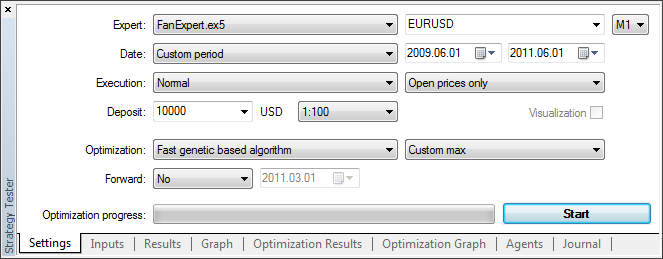Fig. 6. Settings of the strategy tester

The set the range of optimization of input parameters at the Input parameters tab of the strategy tester as is shown in the fig. 7: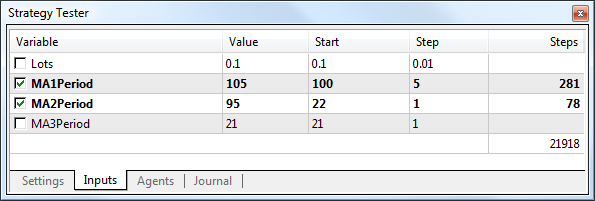Fig. 7. Optimized input parameters

Use the "cloud" agents for the optimization. To do it, set the following parameters at the Agents tab: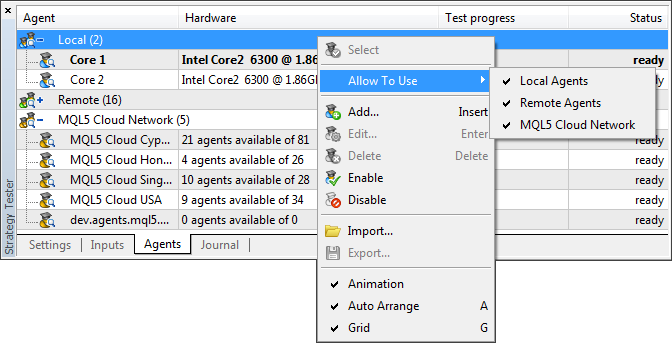Fig. 8. Parameters of agents of testing

Now click the Start button (fig.6) and wait for the optimization to complete. When using the "cloud" calculation technology, the optimization is done pretty fast. In the end, we get the following results of optimization by the specified criteria: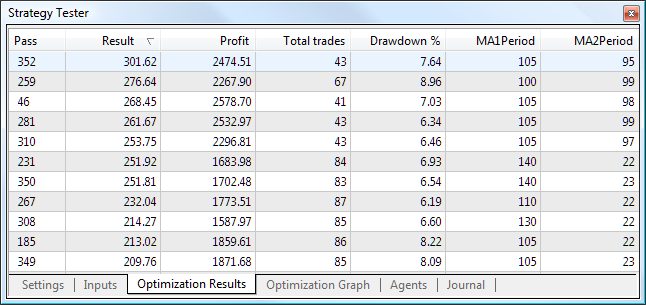Fig. 9. Optimization results

Our "experimental" Expert Advisor has been successfully optimized. It has taken 13 minutes to optimize using the "cloud" agents. The EA for checking this criterion is in the FanExpertSimple.mq5 file attached to the article.

### 8. Creating a Class of a Custom Optimization Criterion on the Basis of Analysis of the Balance Curve

The basis for creation of this class is the "Controlling the Slope of Balance Curve During Work of an Expert Advisor" article. The idea of this optimization criterion is to make the balance line be maximally close to a straight line. The degree of closeness to a straight line will be estimated by the value of standard deviation of trade results from it. The equation of a straight line will be calculated for the regression line drawn by the results of deals in the strategy tester.

To discard curves with negative resulting balance, set additional limits - the resulting profit must be greater than a specified value, and the number of trades must not be less the a specified value.

Thus, our optimization criterion will be inversely proportional to the value of standard deviation of trade results from the straight line considering the limits of the resulting profit and number of trades.

To implement the optimization criterion on the basis of the balance curve we need the TBalanceSlope class from the article mentioned above. We're going to change it: use constructors with parameters (for convenience) and add the calculation of standard deviation to the calculation of the linear regression. This code is located in the BalanceSlope.mqh file attached to the article.

The sequence of steps of adding this optimization criterion to the Expert Advisor is the same as described above. Now, the optimization criteria look as following:

`criterion_Ptr.Add(new TBalanceSlopeCriterion(Symbol( ), 10000.0));`

In addition to the balance curve criterion, we can add other criteria developed by us. For the readers, I leave the possibility to experiment with different sets of statistical parameters of testing.

Let's perform the optimization by the set criteria. To get more trades, perform the optimization using the H4 timeframe, the period 2010.01.01 - 2011.01.01 and the EURUSD symbol. We will get a set of results: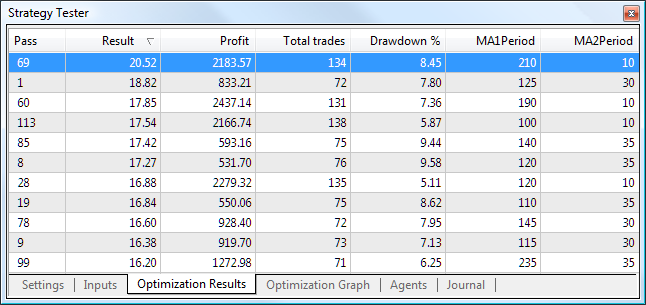Fig. 10. The result of optimization by the balance curve

Now, we need estimate the quality of the optimization. I think that the main criterion is the work of the Expert Advisor outside of the optimization period. To check it, run a single test within the 2010.01.01-2011.06.14 period.

Compare two results (that nearly the same resulting profit) from the set of optimal parameters - the best result with a result from the middle. The results outside the optimization period are separated with the red line: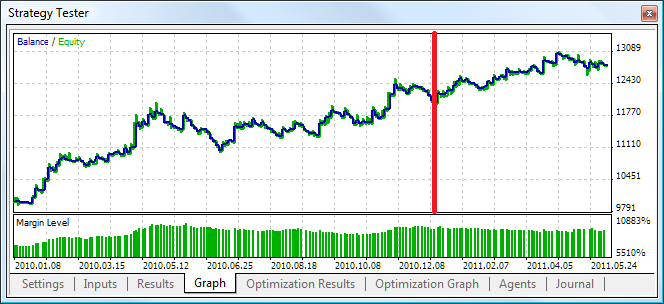Fig. 11. The best result of optimization

Generally, the behavior of the curve hasn't become worse. The profitability has slightly decreased from 1.60 to 1.56.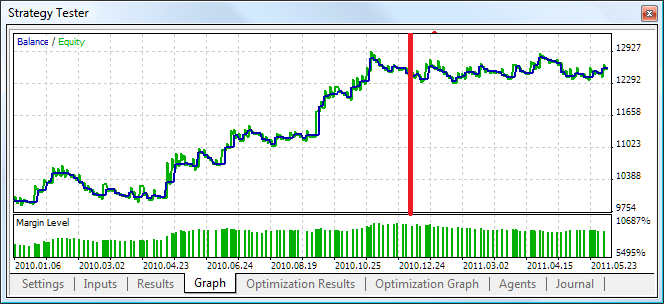Fig. 12. The medium result of testing

The Expert Advisor is not profitable outside the optimization period. The profitability has decreased significantly from 2.17 to 1.75.

Thus, we can make a conclusion that the hypothesis of correlation of the balance curve with the duration of working of the optimized parameters has a right to exist. Certainly, we cannot exclude the variant when an acceptable result of using this criterion is unreachable for an Expert Advisor. In this case, we need to perform some additional analysis and experiments.

Probably, for this criterion we need to use the maximum possible period (but reasonable). The Expert Advisor for checking this criterion is in the FanExpertBalance.mq5 file attached to the article.

### 9. Creating a Class of a Custom Optimization Criterion on the Basis of the Coefficient of the Safe Trade System (CSTS)

As is described in the "Be in-Phase" article, the coefficient of safe trade system (CSTS) is calculated using the following formula:

CSTS = Avg.Win / Avg.Loss ((110% - %Win) / (%Win-10%) + 1)

where:

• Avg.Win - the average value of a profitable deal;
• Avg.Loss - the average value of a losing deal;
• %Win - the percentage of profitable deals;

If the CSTS value is less than 1, the trading system is in the zone of high trade risk; even smaller values indicate the zone of unprofitable trading. The greater is the value of CSTS, the better the trade system fits the market and the profitable it is.

All statistical values required for calculation of CSTS are calculated in the strategy test after each test pass. It is left to create the TTSSFCriterion class inherited from TCustomCriterion and implement the GetCriterion() method in it. The implementation of this method in the code is the following:

```double  TTSSFCriterion::GetCriterion()
{
double  avg_win = TesterStatistics(STAT_GROSS_PROFIT) / TesterStatistics(STAT_PROFIT_TRADES);
double  avg_loss = -TesterStatistics(STAT_GROSS_LOSS) / TesterStatistics(STAT_LOSS_TRADES);

//  Calculated safe ratio for this percentage of profitable deals:
double  teor = (110.0 - win_perc) / (win_perc - 10.0) + 1.0;

//  Calculate real ratio:
double  real = avg_win / avg_loss;

//  CSTS:
double  tssf = real / teor;

return(tssf);
}```

I suppose that short periods are suitable for this criterion of optimization. However, to avoid fitting, we should better take results that are in the middle of results of optimization.

Let's give our readers the possibility to perform optimization on their own. The Expert Advisor for checking this criterion is in the FanExpertTSSF.mq5 file attached to the article.

### Conclusion

Anyway, you must confess that such a simple solution to implementation of possibility of creating custom optimization criteria (using a single integral rate) is almost perfect comparing to other variants. It allows raising the bar of development of robust trade systems to a higher level. Use of the "cloud" technology decreases the limitation of conducted optimizations significantly.

Further ways of evolution may be connected with mathematically and statistically substantiated criteria described in different sources of information. We have a tool for it.

Translated from Russian by MetaQuotes Software Corp.
Original article: https://www.mql5.com/ru/articles/286

Attached files |
balanceslope.mqh (14.56 KB)
fanexpert.mq5 (8.82 KB)
fanexperttssf.mq5 (8.86 KB)

#### Other articles by this author

Last comments | Go to discussion (12)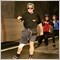| 21 Jan 2014 at 00:36

Well, here I am again, the lone wolf in this universe  :-)

I have been trying the straightness Custom Criteria trying to get the slope of the calculated straight line into the equation. As is it can give you a very hgh rating on a very feeble profit. Just adding the end profit

into the caculation does not make it any better  In an attempt to add the actual slope into the equation  I changed the code lilke seen below.

It is not a perfect solution but it is closer to what I want to see. Using result together wit balance or profit  works fine for me with this code

```//---------------------------------------------------------------------
//      Get value of the optimization result:
//---------------------------------------------------------------------
double  TBalanceSlopeCriterion::GetCriterion()
{
//      Let's try to calculate the slope of the balance curve:
double   current_slope=1000.0*this.balance_Ptr.CalcSlope();

//      If it is inclined down:
if(current_slope<0.0)
{
return(-1.0);
}

double   temp=this.balance_Ptr.GetCurrentSKO();
if(temp>0.0)
{

//   return(this.scale/temp);        //This just returns how well the results adhere to a straight line that could be anything from a level line to a line pointing uppwards
double temp2 = this.scale/temp;   //   added Ingvar

return(temp2 * current_slope);    // added Ingvar

}

return(0.0);
}```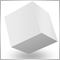| 21 Jan 2014 at 01:39
ingvar_e:

Well, here I am again, the lone wolf in this universe  :-)

I have been trying the straightness Custom Criteria trying to get the slope of the calculated straight line into the equation. As is it can give you a very hgh rating on a very feeble profit. Just adding the end profit

into the caculation does not make it any better  In an attempt to add the actual slope into the equation  I changed the code lilke seen below.

It is not a perfect solution but it is closer to what I want to see. Using result together wit balance or profit  works fine for me with this code

ingvar_e, not so alone, I like too much this area and was surprised with your evolution, because I do believe in this line of thought too.

For instance, for genetic algorithms, you must have a good fitness algorithm, and for sure create an custom criteria aligned to this fitness.

I'm not sure calculate the slope of balance curve is the better way, since we have several other ways, anyway count on me to explore and debate this ideas.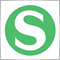| 21 Feb 2014 at 11:15

With much experimenting, I have come to similar conclusions. I don't know why it took me so long to find this article and discussion.

I started with a problem where my break out and trend riding strategies tended to fit themselves to a few super profitable trades. So much profit from these few that the optimizer only "cared" about these few trades and used wide stops and far away take profit and making sure its signal caught and milked these few trades to the maximum. this occurred even on 20 year samples with 100's of trades. Even when optimizing with a dd to profit ratio, it still went after the runs with these trades at all costs cause the runs were 90% of its profit. I wanted to limit this behavior in some way without cutting the head off of the breakout/trend ride strat. I found that optimizing for relative dd percent (NOT dd ratio to profit, just dd relative percent only and nothing much else) gives good results going forward as long as minimum trades and minimum profit factor (about 1.3) are met.

```//...............

sinput double mint; //minimum trades for optimization purposes. Will impose a penalty on runs with fewer trades.

sinput double minpf; //minimum profit factor for optimization purposes. Will impose a penalty on runs with less pf.

//...............

double OnTester()

{

double dd=TesterStatistics(STAT_BALANCE_DDREL_PERCENT);//equity instead of balance also worked well going forward

double pf=TesterStatistics(STAT_PROFIT_FACTOR);

double custom=(100-dd);

if (mint>t) custom=custom*(tt/mint); // imposes a penalty if minimum trades not met

if (minpf>minpf) custom=custom*((pf-1)/(minpf-1)) // this line also causes losing runs to be negative as well as imposing a penalty lesser pf.

return(custom);

}

```

It seems wrong to not have profit involved but by having a pf minimum criteria, mostly quite profitable runs are appearing at the top. Cropping out dd from profitable runs is usually increasing profits. In any case, by selecting the more profitable trades and doing the final touch-ups carefully manually by hand (such as near the end of this excellent article https://www.mql5.com/en/articles/156), I can allow the optimizer to do a lot of the initial work while avoiding a lot of curve fitting. Ive experimented and had mixed results with code such as the following. (Experiments should include changing risk and starting balance too once you begin put additional weights in on top of a percent based score)

```//.............

sinput double pfw //Profit factor weight

//.............

double custom=(100-dd)+(pf-1)*pfw;
//...........
```| 2 Feb 2019 at 17:02

I have tested Kelly Criterion (Strategy), using the following code:

```double OnTester(void)
{
double Kelly=(r!=0)?w-((1-w)/r):0; // Kelly Criterion
return(Kelly);
}```

I am not sure if Metatrader Strategy Tester computes 0 (zero) profit trades as profit trades. Anyone?| 22 Mar 2020 at 04:39
Ingvar Engelbrecht:

Well, here I am again, the lone wolf in this universe  :-)

I have been trying the straightness Custom Criteria trying to get the slope of the calculated straight line into the equation. As is it can give you a very hgh rating on a very feeble profit. Just adding the end profit

into the caculation does not make it any better  In an attempt to add the actual slope into the equation  I changed the code lilke seen below.

It is not a perfect solution but it is closer to what I want to see. Using result together wit balance or profit  works fine for me with this code

I know it's been so long since you posted this but in case you are still playing with this or someone else are looking for the same straightness criteria implementation.

I found a working public solution here https://community.darwinex.com/t/equity-curve-straigthness-optimization-with-metatrader/3976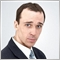Andrey Voitenko (avoitenko): &quot;Developers benefit from the ideas that they code? Nonsense!&quot;
A Ukrainian developer Andrey Voitenko (avoitenko) is an active participant of the "Jobs" service at mql5.com, helping traders from all over the world to implement their ideas. Last year Andrey's Expert Advisor was on the fourth place in the Automated Trading Championship 2010, being slightly behind the bronze winner. This time we are discussing the Jobs service with Andrey.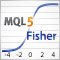Applying The Fisher Transform and Inverse Fisher Transform to Markets Analysis in MetaTrader 5
We now know that probability density function (PDF) of a market cycle does not remind a Gaussian but rather a PDF of a sine wave and most of the indicators assume that the market cycle PDF is Gaussian we need a way to "correct" that. The solution is to use Fisher Transform. The Fisher transform changes PDF of any waveform to approximately Gaussian. This article describes the mathematics behind the Fisher Transform and the Inverse Fisher Transform and their application to trading. A proprietary trading signal module based on the Inverse Fisher Transform is presented and evaluated.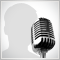Andrey Bolkonsky (abolk): "Any programmer knows that there is no software without bugs"
Andrey Bolkonsky (abolk) has been participating in the Jobs service since its opening. He has developed dozens of indicators and Expert Advisors for the MetaTrader 4 and MetaTrader 5 platforms. We will talk with Andrey about what a server is from the perspective of a programmer.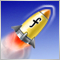3 Methods of Indicators Acceleration by the Example of the Linear Regression
The article deals with the methods of indicators computational algorithms optimization. Everyone will find a method that suits his/her needs best. Three methods are described here.One of them is quite simple, the next one requires solid knowledge of Math and the last one requires some wit. Indicators or MetaTrader5 terminal design features are used to realize most of the described methods. The methods are quite universal and can be used not only for acceleration of the linear regression calculation, but also for many other indicators.